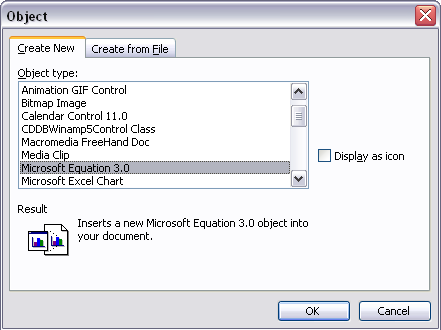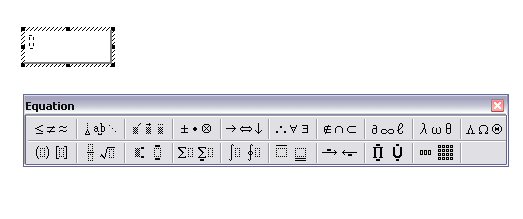# Mathematical symbols in microsoft word/ms word(set theory symbols,integral symbols)

#### by Ramya 2008-10-13 11:02:25

Mathematical symbols in word:

To use mathematical symbols in word,

> Go to Insert->Object.

> Select "Microsoft Equation 3.0".> "Equation" window will open.> Select the symbol you want for the equation.

* Relational symbols
* Spaces and ellipses
* Embellishments
* Operator symbols
* Arrow symbols
* Logical symbols
* Set theory symbols
* Integral symbols